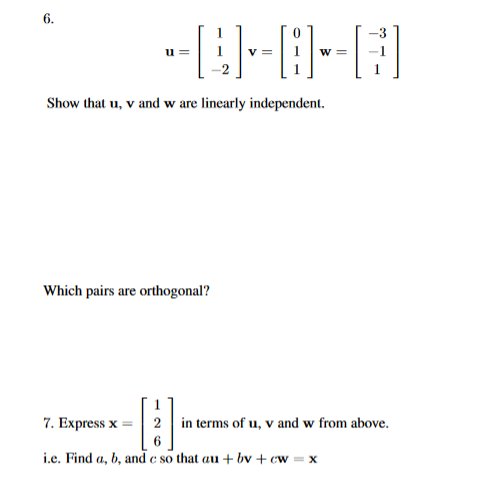3 8-1UDIShow that u, v and w are linearly independent.Which pairs are orthogonal?7. Express x2in terms of u, v and w from above.i.e. Find a, b, and c so that aubv +ewx

Question

Question 7help_outlineImage Transcriptionclose3 8-1 UDI Show that u, v and w are linearly independent. Which pairs are orthogonal? 7. Express x2in terms of u, v and w from above. i.e. Find a, b, and c so that au bv +ewx fullscreen
Step 1

Every element of the vector space can be written as linear combination of the linear independent elements.

Step 2

Simplify the above three equation and obtain the value of given variable.

Step 3

compute the variables  in equation and the vector can be writt...

Want to see the full answer?

See Solution

Want to see this answer and more?

Our solutions are written by experts, many with advanced degrees, and available 24/7

See Solution
Tagged in

Vectors